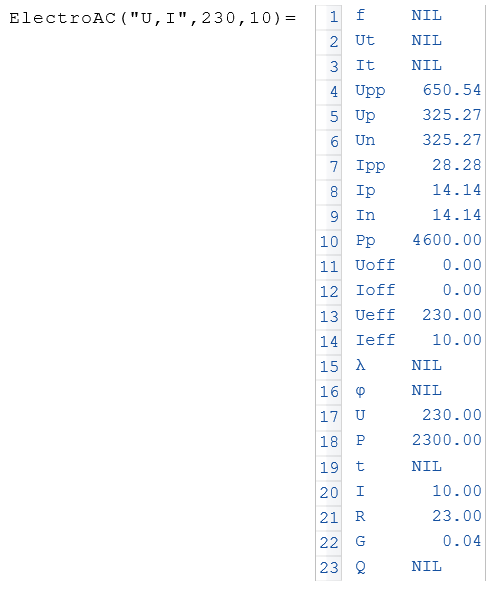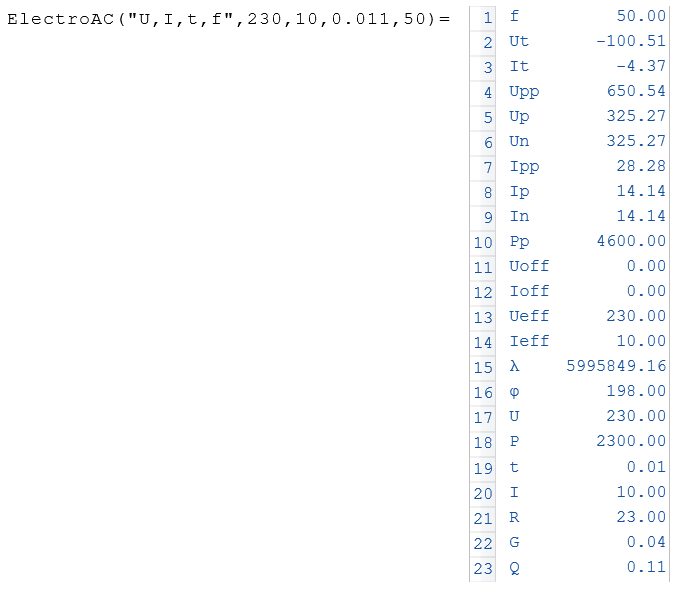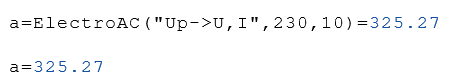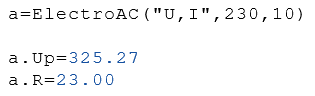# Class ElectroAC

Calculation of AC voltage and current properties

## Description

The class $$ElectroAC$$ contains methods for the calculation of AC voltage and current properties. This class is an extension of the class Electro. This means that all properties and methods of the class Electro are also included in this class.

## Properties

#### Description

U

AC voltage in Volts

I

Alternating current in Amperes

P

Electrical power in Watts

R

Resistance in Ohms

G

Conductance in Siemens

Q

Charge in Coulomb

Ueff

Effective AC voltage
Considered a superimposed DC voltage

Upp

Peak voltage from peak to peak

Up

Positive peak voltage

Un

Negative peak voltage

Ieff

Effective AC
Considered a superimposed DC current

Ipp

Peak current peak to peak

Ip

Positive peak current

In

Negative peak current

Pp

Peak power

Uoff

Superimposed DC (offset voltage)

Ioff

Superimposed DC current (current offset)

t

Time in Seconds
Time to calculate $$Ut$$ or $$It$$

f

Frequency in Hertz

λ

Wave length in Meters

Ut

Voltage at a specific time

It

Current at any given time

phi - φ

Phase angle in degrees at the specific time t

## Examples

Example 1Example 2Example 3Example 4